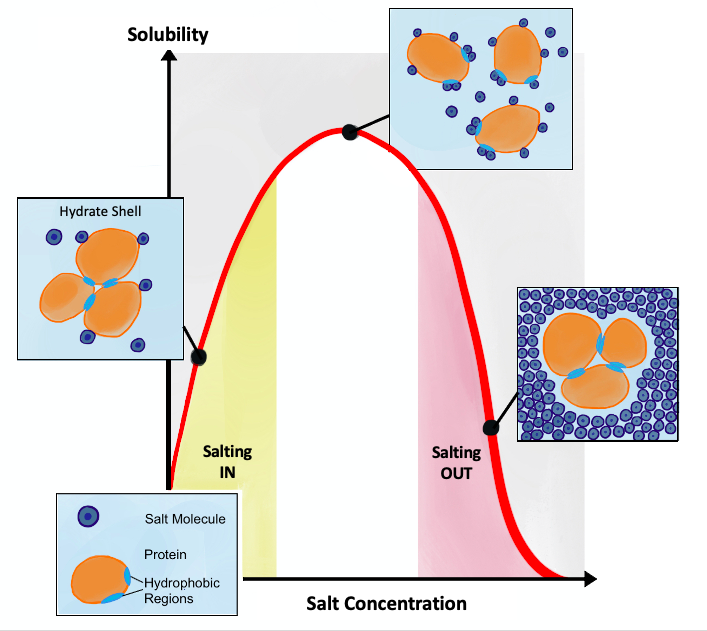# Carbon-dioxide solubility in brines with different salinity and temperature#

Written by Svetlana Kyas (ETH Zurich) on Mar 30th, 2022

Attention

Always make sure you are using the latest version of Reaktoro. Otherwise, some new features documented on this website will not work on your machine and you may receive unintuitive errors. Follow these update instructions to get the latest version of Reaktoro!

Salting out is usually used to precipitate large biomolecules such as proteins or DNA. A specific salt concentration can be used to precipitate a target protein. This method is also used to concentrate dilute solutions of proteins. If necessary, the salt can be removed by dialysis.Salting in vs. salting out, Source: wikipedia.org

This tutorial shows how to simulate the solubility of CO2 gas in NaCl brine and its dependence on brine salinity (also called the salting-out effect) and temperature. To perform such a study, we need a chemical system consisting of an aqueous and a gaseous phase. The corresponding equilibrium solvent will also be initialized in the following.

from reaktoro import *

# Initialize a thermodynamic database
db = PhreeqcDatabase("phreeqc.dat")

# Create an aqueous phase automatically selecting all species with provided elements
aqueousphase = AqueousPhase(speciate("H O C Na Cl"))
aqueousphase.set(ActivityModelPhreeqc(db))

# Create a gaseous phase with CO2(g)
gaseousphase = GaseousPhase("CO2(g)")
gaseousphase.set(ActivityModelPengRobinsonPhreeqcOriginal())

# Create the chemical system
system = ChemicalSystem(db, aqueousphase, gaseousphase)

# Create the equilibrium solver
solver = EquilibriumSolver(system)


Next, the temperature and salinity ranges, as well as the pressure for the calculation of the CO2 solubilities, are defined. The resulting pandas.DataFrame contains the amount of carbon dissolved in the aqueous solution as a function of the respective temperature and NaCl concentration.

import numpy as np
import pandas as pd

temperatures = np.arange(25.0, 90.0, 5.0)
molsNaCl  = np.array([1.0, 2.0, 4.0])
P = 100.0

df = pd.DataFrame(columns=["T", "amountNaCl", "amountCaq"])


In a loop, we perform equilibrium calculations for different input temperatures and different brine concentrations.

for molNaCl in molsNaCl:
for T in temperatures:

# Initial amount of the CO2 gas
n0CO2g = 10.0

# Define initial chemical state corresponding to the NaCl-brine of the given concentration
state = ChemicalState(system)
state.setTemperature(T, "celsius")
state.setPressure(P, "bar")
state.set("H2O"   , 1.0   , "kg")
state.set("CO2(g)", n0CO2g, "mol")
state.set("Na+"   , molNaCl , "mol")
state.set("Cl-"   , molNaCl , "mol")

# Calculate equilibrium state
res = solver.solve(state)
# Stop if the equilibration did not converge or failed
if not res.succeeded(): continue

# Fetch resulting aqueous properties of the chemical state
aqprops = AqueousProps(state)

# Update value ["T", "amountNaCl", "amountCaq"] in the dataframe
df.loc[len(df)] = [T, molNaCl, float(aqprops.elementMolality("C"))]


Below, we plot the solubility of CO2(g) as a function of temperature for NaCl brines with different salinities.

from reaktplot import *

fig = Figure()
fig.title("SOLUBILITY OF CO2 IN NACL BRINES")
fig.xaxisTitle('TEMPERATURE [°C]')
fig.yaxisTitle('AMOUNT OF DISSOLVED CO2 [mol/kgw]')

for molNaCl in molsNaCl:
df_NaCl = df[df['amountNaCl'] == molNaCl]
fig.drawLineWithMarkers(df_NaCl["T"], df_NaCl["amountCaq"], name=f'{molNaCl} mol of NaCl')

fig.show()